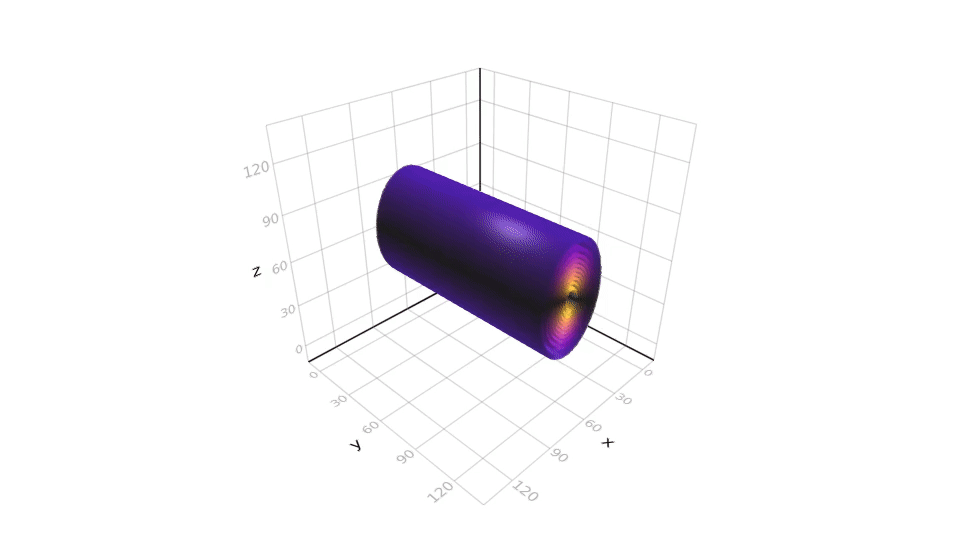Julia & graphic enthusiast. Interested in high performance computing, GPUs and machine learning.

# Oceananigans

# install additional packages
# make sure everything is installed + compiled
using Oceananigans, CuArrays, Makie
cuzeros(Float32, 32, 32) .+ cuzeros(Float32, 32, 32);
# export runtime & lock cell to not be run again and import this new docker image in cell below:;
272.4s
Julia
Julia 1.1 Oceananigans
using Oceananigans, CuArrays, Makie
# We'll set up a 2D model with an xz-slice so there's only 1 grid point in y.
Nx, Ny, Nz = 128, 128, 128     # Number of grid points in each dimension.
Lx, Ly, Lz = 2000, 2000, 2000  # Domain size (meters).
Nt, Δt = 5000, 50              # Number of time steps, time step size (seconds).

# Set up the model and use an artificially high viscosity ν and diffusivity κ.
model = Model(N=(Nx, Ny, Nz), L=(Lx, Ly, Lz), arch=GPU(), ν=4e-2, κ=4e-2)

# Get location of the cell centers in x, y, z and reshape them to easily
# broadcast over them when calculating hot_bubble_perturbation.
xC, yC, zC = model.grid.xC, model.grid.yC, model.grid.zC
xC, yC, zC = reshape(xC, (Nx, 1, 1)), reshape(yC, (Ny, 1, 1)), reshape(zC, (1, 1, Nz))

# Set a temperature perturbation with a Gaussian profile located at the center
# of the vertical slice. It roughly corresponds to a background temperature of
# T = T₀ [°C] and a bubble temperature of T = T₀ + 0.01 [°C] where T₀ is the
# reference temperature in the equation of state (eos).
hot_bubble_perturbation = @. 0.01 * exp(-100 * ((xC - Lx/2)^2 + (yC - Ly/2)^2 + (zC + Lz/2)^2) / (Lx^2 + Ly^2 + Lz^2))
data(model.tracers.T) .= model.eos.T₀ .- 0.01 .+ 2 .* CuArray(hot_bubble_perturbation)

temp = cuzeros(Float32, size(data(model.tracers.T)))
scene = contour(
collect(temp), levels = 10, alpha = 0.6, colormap = :plasma
)
vplot = scene[end]
vplot.transformation.flip[] = (false, true, true)
record(scene, "/results/sim.gif", 1:1000) do i
@show i
time_step!(model, 1, Δt)
xx = data(model.tracers.T);
temp .= xx
vplot = collect(temp)
end
"/results/sim.gif"